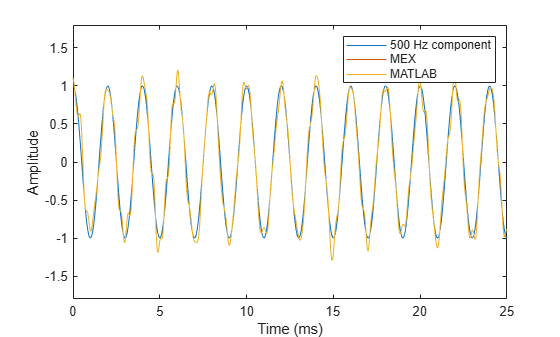# Zero-Phase Filtering

Design a lowpass Butterworth filter with a 1 kHz 3-dB frequency to implement zero-phase filtering on data sampled at a rate of 20 kHz.

`type myZerophaseFilt.m`
```function output = myZerophaseFilt(input) %#codegen [B,A] = butter(20,0.314); output = filtfilt(B,A,input); end ```

Use codegen to create the MEX file for `myZerophaseFilt.m`.

`codegen myZerophaseFilt -args {zeros(1,20001)} -o myZerophaseFilt_mex -report`
```Code generation successful: To view the report, open('codegen/mex/myZerophaseFilt/html/report.mldatx') ```

Generate a noisy sinusoid signal as input to the filter.

```Fs = 20000; t = 0:1/Fs:1; comp500Hz = cos(2*pi*500*t); signal = comp500Hz + sin(2*pi*4000*t) + 0.2*randn(size(t));```

Filter input data using both MATLAB® and MEX functions.

```FilteredData = myZerophaseFilt(signal); MexFilteredData = myZerophaseFilt_mex(signal);```

Plot the 500 Hz component and the filtered data.

```tms = t*1000; plot(tms,comp500Hz) hold on plot(tms,MexFilteredData) plot(tms,FilteredData) hold off xlabel('Milliseconds') ylabel('Amplitude') axis([0 25 -1.8 1.8]) legend('500 Hz component','MEX','MATLAB')```